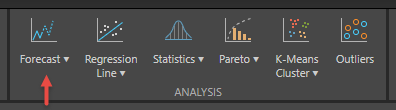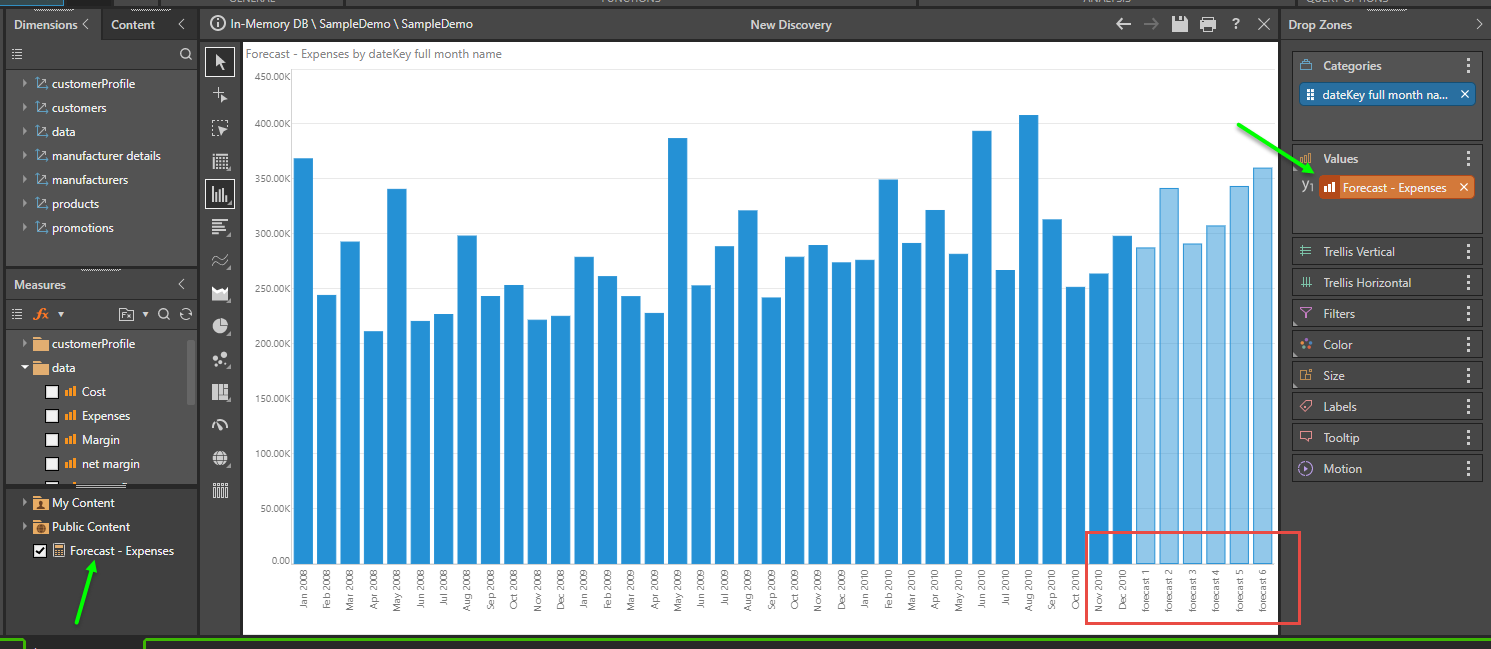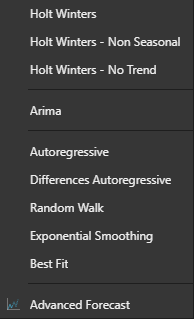# Basic Forecasting

Basic forecasting allows you to attach one of the built-in forecasting models to your query without opening the Advanced Forecasting dialog. Basic forecasting is the quickest and easiest method of attaching a forecasting model to your query.

To add a forecast to your query and visualization, simply click on the top part of the forecast button in the 'Analysis' button group of the Query tab.This will apply the default forecasting 'solution' to your dataset. The solution employs the 'best fit' model option which runs all the pre-configured forecasting models in Pyramid (see below), and selects the model that produces the best result statistically.

#### The Forecast Value Chip

The process of adding a forecasting actually materializes a new custom measure or orange value chip into your Discover session. This chip holds the logic to represent the forecasted values. Since its a simple measure chip, you can move it around the drop-zones and visualizations freely like any other metric. This will also allow users to show forecasted values side-by-side with other metrics; in trellised charts; as secondary axis measures; as items in a measure set; or even as a measure to drive color and size logic.

• The forecast chip is described in more detail in the overview.### Specific Model Selection

As described above, the default forecast uses the 'best fit' solution. Alternatively, you can elect to run a specific forecast model by choosing from the drop list (by clicking the bottom part of the forecast button).### Forecasting Models

• Holt Winters: forecasting model which includes exponential smoothing with modeling for time series has both a trend and seasonality.
• Holt Winters - Non Seasonal: same as Holt-Winters but assumes there isn’t a seasonal factor in the data.
• Holt Winters - No Trend: same as Holt-Winters but assumes there isn’t a trend factor in the data.
• Arima: forecasting model with Auto Regressive Integrated Moving Average for data with stationary time series and for data that may contain seasonality.
• It models the time series data by looking at the differences between the adjacent data points (assuming those differences have a trend), and random errors (white noises). Also assumes an additional seasonal factor which also has (in its differences) a trend and random errors.
• The default Arima algorithm runs a few combinations of the parameters and chooses the best ones for the given data. In the Advanced panel it is possible to control how many historical data points are used for predicting the next data point.
• Autoregressive: models the time series data assuming the next value depends on the previous values.
• Differences Autoregressive: models the time series data assuming that the difference between the next value and the current value depends on the differences between adjacent historical points.
• Exponential Smoothing: Forecasting model with a Simple Moving Average (SMA). Models the time series data assuming the next data point depends on the previous data points, such that the further into the past a data point is, the less it affects the value of the next data point.
• Best Fit (default): hides some data points at the end of the time series, then creates various forecasting models and chooses the one which best predicts the hidden points, and reconstructs the best model without hiding the data points at the end.

IMPORTANT: The Standard and Seasonal forecasting models cannot be run on years. These two models can be run on quarters, months and days only. The reason is that these two models employ seasonality (gamma) which cannot be calculated in time periods of years.